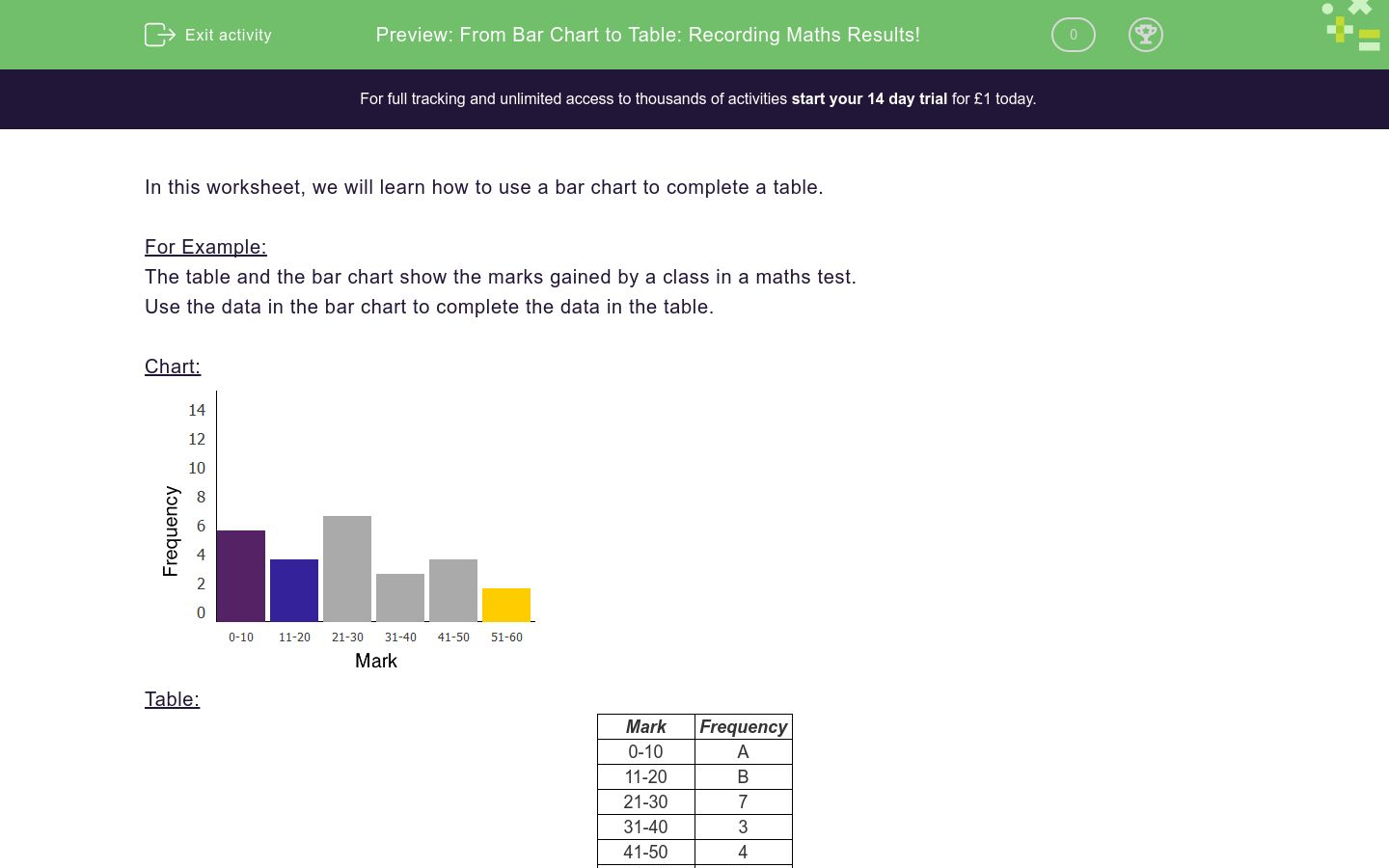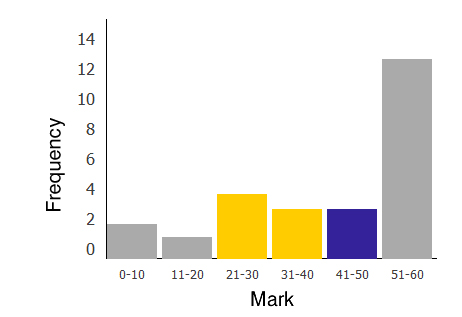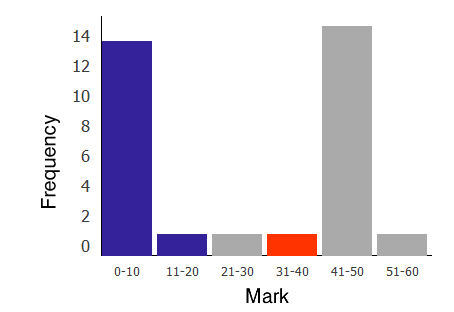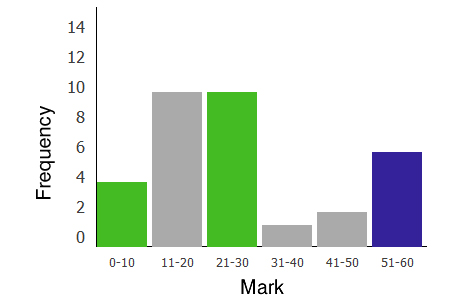# From Bar Chart to Table: Recording Maths Results!

In this worksheet, students use the data on a bar chart to put into a table.Key stage:  KS 2

Curriculum topic:   Statistics

Curriculum subtopic:   Use Charts and Graphs

Difficulty level:### QUESTION 1 of 10

In this worksheet, we will learn how to use a bar chart to complete a table.

For Example:

The table and the bar chart show the marks gained by a class in a maths test.
Use the data in the bar chart to complete the data in the table.

Chart:Table:

Mark Frequency
 0-10
 A
 11-20
 B
 21-30
 7
 31-40
 3
 41-50
 4
 51-60
 C

Find A, B, C.

How many children took the test?

A = 6,

B = 4,

C = 2

Number of children who took the test = 6+4+7+3+4+2 = 26

Complete the table using the bar chart showing the marks gained by a class in a maths test.

Chart:Table:

Mark Frequency
 0-10
 A
 11-20
 B
 21-30
 12
 31-40
 4
 41-50
 C
 51-60
 2

Match up A, B and C with the correct answers below.

## Column B

A
2
B
4
C
4

Complete the table using the bar chart showing the marks gained by a class in a maths test.

Chart:Table:

Mark Frequency
 0-10
 A
 11-20
 10
 21-30
 8
 31-40
 1
 41-50
 B
 51-60
 C

Match up A, B and C with the correct answers below.

## Column B

A
1
B
1
C
6

Complete the table using the bar chart showing the marks gained by a class in a maths test.

Chart:Table:

Mark Frequency
 0-10
 2
 11-20
 1
 21-30
 A
 31-40
 B
 41-50
 C
 51-60
 13

Find A, B, C.

Complete the table using the bar chart showing the marks gained by a class in a maths test.

Chart:Table:

Mark Frequency
 0-10
 A
 11-20
 B
 21-30
 1
 31-40
 C
 41-50
 15
 51-60
 1

Find A, B, C.

Complete the table using the bar chart showing the marks gained by a class in a maths test.

Chart:Table:

Mark Frequency
 0-10
 A
 11-20
 10
 21-30
 B
 31-40
 1
 41-50
 2
 51-60
 C

Find A, B, C.

Complete the table using the bar chart showing the marks gained by a class in a maths test.

Chart:Table:

Mark Frequency
 0-10
 6
 11-20
 A
 21-30
 4
 31-40
 B
 41-50
 C
 51-60
 5

Find A, B, C.

Complete the table using the bar chart showing the marks gained by a class in a maths test.

Chart:Table:

Mark Frequency
 0-10
 A
 11-20
 B
 21-30
 C
 31-40
 7
 41-50
 2
 51-60
 6

Find A, B, C.

Complete the table using the bar chart showing the marks gained by a class in a maths test.

Chart:Table:

Mark Frequency
 0-10
 A
 11-20
 B
 21-30
 12
 31-40
 4
 41-50
 C
 51-60
 2

How many students took the test?

Complete the table using the bar chart showing the marks gained by a class in a maths test.

Chart:Table:

Mark Frequency
 0-10
 A
 11-20
 10
 21-30
 8
 31-40
 1
 41-50
 B
 51-60
 C

How many students took the test?

Complete the table using the bar chart showing the marks gained by a class in a maths test.

Chart:Table:

Mark Frequency
 0-10
 A
 11-20
 B
 21-30
 C
 31-40
 7
 41-50
 2
 51-60
 6

How many students took the test?

• Question 1

Complete the table using the bar chart showing the marks gained by a class in a maths test.

Chart:Table:

Mark Frequency
 0-10
 A
 11-20
 B
 21-30
 12
 31-40
 4
 41-50
 C
 51-60
 2

Match up A, B and C with the correct answers below.

## Column B

A
2
B
4
C
4
EDDIE SAYS
The frequency chart shows that A is 2, B is 4 and C is 4.
• Question 2

Complete the table using the bar chart showing the marks gained by a class in a maths test.

Chart:Table:

Mark Frequency
 0-10
 A
 11-20
 10
 21-30
 8
 31-40
 1
 41-50
 B
 51-60
 C

Match up A, B and C with the correct answers below.

## Column B

A
6
B
1
C
1
EDDIE SAYS
The frequency chart shows that A is 6, B is 1 and C is 1.
• Question 3

Complete the table using the bar chart showing the marks gained by a class in a maths test.

Chart:Table:

Mark Frequency
 0-10
 2
 11-20
 1
 21-30
 A
 31-40
 B
 41-50
 C
 51-60
 13

Find A, B, C.

EDDIE SAYS
The frequency chart shows that A is 4, B is 3 and C is 3.
• Question 4

Complete the table using the bar chart showing the marks gained by a class in a maths test.

Chart:Table:

Mark Frequency
 0-10
 A
 11-20
 B
 21-30
 1
 31-40
 C
 41-50
 15
 51-60
 1

Find A, B, C.

EDDIE SAYS
The frequency chart shows that A is 14, B is 1 and C is 1.
• Question 5

Complete the table using the bar chart showing the marks gained by a class in a maths test.

Chart:Table:

Mark Frequency
 0-10
 A
 11-20
 10
 21-30
 B
 31-40
 1
 41-50
 2
 51-60
 C

Find A, B, C.

EDDIE SAYS
The frequency chart shows that A is 4, B is 10 and C is 6.
• Question 6

Complete the table using the bar chart showing the marks gained by a class in a maths test.

Chart:Table:

Mark Frequency
 0-10
 6
 11-20
 A
 21-30
 4
 31-40
 B
 41-50
 C
 51-60
 5

Find A, B, C.

EDDIE SAYS
The frequency chart shows that A is 1, B is 10 and C is 1.
• Question 7

Complete the table using the bar chart showing the marks gained by a class in a maths test.

Chart:Table:

Mark Frequency
 0-10
 A
 11-20
 B
 21-30
 C
 31-40
 7
 41-50
 2
 51-60
 6

Find A, B, C.

EDDIE SAYS
The frequency chart shows that A is 1, B is 8 and C is 1.
• Question 8

Complete the table using the bar chart showing the marks gained by a class in a maths test.

Chart:Table:

Mark Frequency
 0-10
 A
 11-20
 B
 21-30
 12
 31-40
 4
 41-50
 C
 51-60
 2

How many students took the test?

28
EDDIE SAYS
Add up the number of students who took the test based on the frequency chart. 2 + 4 + 12 + 4 + 4 + 2 = 28
• Question 9

Complete the table using the bar chart showing the marks gained by a class in a maths test.

Chart:Table:

Mark Frequency
 0-10
 A
 11-20
 10
 21-30
 8
 31-40
 1
 41-50
 B
 51-60
 C

How many students took the test?

29
EDDIE SAYS
Add up the number of students who took the test based on the frequency chart. 6 + 10 + 8 + 1 + 2 + 2 = 29
• Question 10

Complete the table using the bar chart showing the marks gained by a class in a maths test.

Chart:Table:

Mark Frequency
 0-10
 A
 11-20
 B
 21-30
 C
 31-40
 7
 41-50
 2
 51-60
 6

How many students took the test?

25
EDDIE SAYS
Add up the number of students who took the test based on the frequency chart. 1 + 8 + 1 + 7 + 2 + 6 = 25
---- OR ----

Sign up for a £1 trial so you can track and measure your child's progress on this activity.

### What is EdPlace?

We're your National Curriculum aligned online education content provider helping each child succeed in English, maths and science from year 1 to GCSE. With an EdPlace account you’ll be able to track and measure progress, helping each child achieve their best. We build confidence and attainment by personalising each child’s learning at a level that suits them.

Get started